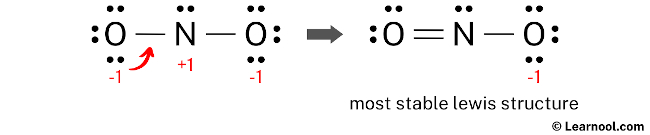# Lewis structure of NO2-

The Lewis structure of NO2 represents the molecular arrangement of nitrite, which consists of one nitrogen atom and two oxygen atoms. The structure shows one double bond and one single bond around the nitrogen atom, with two oxygen atoms attached to it. The oxygen atom involved in the double bond has two lone pairs, while the oxygen atom involved in the single bond has three lone pairs. The nitrogen atom, on the other hand, has one lone pair. Additionally, there is a negative charge (-1) on the oxygen atom involved in the single bond.

To accurately draw the Lewis structure of NO2, start by sketching a rough skeleton structure of the molecule. Then, indicate any lone pairs of electrons on the atoms within the structure. Next, determine and mark the formal charges on the atoms if needed. Proceed to minimize the formal charges by adjusting lone pairs of electrons, converting them into double or triple bonds when necessary, in order to achieve a stable Lewis structure with reduced charges. Repeat this process as needed until all charges are minimized. By following these systematic steps, one can accurately depict the Lewis structure of NO2.

Contents

## Steps

### Sketch the structure

The first step in drawing the Lewis structure of NO2 is to determine the total number of valence electrons. The total valence electrons in the molecule can be calculated by multiplying the valence electrons of each atom. NO2 molecule consists of one nitrogen atom and two oxygen atoms. Nitrogen, which belongs to group 15, has five valence electrons, while oxygen, belonging to group 16, has six valence electrons. Considering the composition of NO2, the valence electrons for one nitrogen atom are 5, and for two oxygen atoms, it’s 6 each, totaling 12. Additionally, taking into account the -1 charge on NO2, one more electron needs to be included in the count. Therefore, the total number of valence electrons for NO2 is 5 + 12 + 1 = 18.

After determining the total number of valence electrons, the next step in sketching the Lewis structure of NO2 is to determine the total electron pairs. This can be accomplished by dividing the total number of valence electrons by two. In the case of NO2 with 18 valence electrons, the total electron pairs would be obtained by dividing 18 by 2, resulting in 9 electron pairs.

Once the electron pairs have been determined, the next step is to identify the central atom. It is advisable to position the atom with the lowest electronegativity at the center. In the case of the NO2 molecule, nitrogen exhibits lower electronegativity than oxygen, making nitrogen the preferred choice as the central atom. Taking this into consideration, the rough sketch for the Lewis structure of NO2 can now be drawn.

### Indicate lone pair

After sketching the rough structure, the next step in drawing the Lewis structure of NO2 is to indicate the lone pairs. There are a total of 9 electron pairs, where two electron pairs are already used for the N – O bonds that are marked. Therefore, the remaining seven electron pairs should be marked as lone pairs on the sketch.

Also, it is important to remember that both nitrogen and oxygen are period 2 elements, which means they cannot accommodate more than 8 electrons in their outermost shell.

When marking lone pairs, it is important to begin with the outermost atoms. In this molecule, the outer atoms are the two oxygen atoms. Each oxygen atom will have three lone pairs assigned to it, while nitrogen will have one lone pair. Mark the lone pairs on the sketch as discussed, ensuring the appropriate distribution.

### Assign formal charge

To determine the formal charges on the atoms in NO2, apply the formula: Formal charge = valence electrons – nonbonding electrons – ½ bonding electrons. For the nitrogen atom, the formal charge is calculated as 5 – 2 – ½ (4) = +1. Likewise, for each oxygen atom, the formal charge is obtained by the calculation 6 – 6 – ½ (2) = -1.

 Atom Valence electron Nonbonding electron Bonding electron Formal charge Nitrogen 5 2 4 +1 Oxygen 6 6 2 -1

After calculating the formal charges for both nitrogen and oxygen atoms, it is evident that they possess charges. Therefore, proceed to mark these charges on the sketch accordingly.

The existing Lewis structure of NO2 is deemed unstable due to the presence of charges on both the nitrogen and oxygen atoms. Therefore, in the subsequent step, it is crucial to mitigate these charges by converting lone pairs into bonds.

### Minimize formal charge

In order to minimize the formal charges on atoms, it is possible to convert a lone pair of the oxygen atom into a new N – O bond with the nitrogen atom.Lone pair of left oxygen is converted, and got the most stable Lewis structure of NO2–

The final Lewis structure of NO2 demonstrates the fulfillment of the octet rule, with the central nitrogen atom and the surrounding oxygen atoms forming complete octets. Therefore, the octet rule is satisfied in this structure. However, there remains a negative charge (-1) on one of the oxygen atoms. This is acceptable since the Lewis structure with a negative charge on the most electronegative atom, which is oxygen in this case, is considered the most favorable. Furthermore, this structure exhibits greater stability compared to the previous structures, making it the most stable Lewis structure for NO2. To accurately represent the negative charge (-1) in NO2, brackets should be drawn around the Lewis structure, with (-1) written in the top right corner.